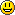#创客百科

### 侧边栏

• 树莓派瑞士军刀扩展板

Arduino

MicroPython

micro:bit

en:arduino:language:bitwisecompoundor

# compound bitwise OR (|=)

## Description

The compound bitwise OR operator (|is often used with a variable and a constant to “set” (set to 1) particular bits in a variable.

## Syntax:

`x |= y;   // equivalent to x = x | y; `

## Parameters

x: a char, int or long variable

y: an integer constant or char, int, or long

## Example:

First, a review of the Bitwise OR (|) operator

```   0  0  1  1    operand1
0  1  0  1    operand2
----------
0  1  1  1    (operand1 | operand2) - returned result```

Bits that are “bitwise ORed” with 0 are unchanged, so if myByte is a byte variable,

`myByte | B00000000 = myByte;`

Bits that are “bitwise ORed” with 1 are set to 1 so:

`myByte | B11111111 = B11111111;`

Consequently - to set bits 0 & 1 of a variable, while leaving the rest of the variable unchanged, use the compound bitwise AND operator (|with the constant B00000011

```   1  0  1  0  1  0  1  0    variable
0  0  0  0  0  0  1  1    mask
----------------------
1  0  1  0  1  0  1  1

variable unchanged
bits set```

Here is the same representation with the variables bits replaced with the symbol x

```   x  x  x  x  x  x  x  x    variable
0  0  0  0  0  0  1  1    mask
----------------------
x  x  x  x  x  x  1  1

variable unchanged
bits set```

So if:

```myByte =  B10101010;

myByte |= B00000011 == B10101011;```

en/arduino/language/bitwisecompoundor.txt · 最后更改: 2016/12/25 22:15 (外部编辑)

### 页面工具